# Tensorflow Data Validation

Posted by ChenRiang on May 29, 2021

In Google’s whitepaper (“Hidden Technical Debt in Machine Learning Systems”) highlighted that in a real production system, machine learning(ML) actually is just the tips of iceberg. To create a efficient and enterprise grade ML system, the required surrounding infrastructure is vast and complex.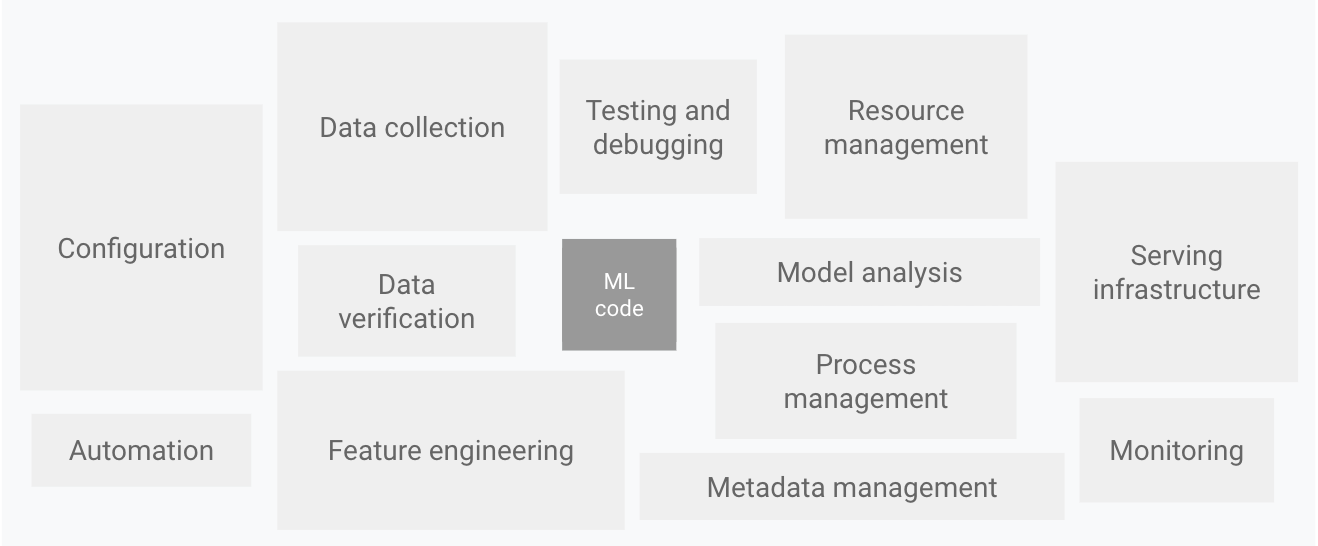To solve this problem, Google actually come out a library called TensorFlow Extended (TFX) which help data scientist / developer to create a scalable and high-performance ML pipeline. However, the focus of this article will be data validation and hence we will look into one of the component in TFX called TensorFlow Data Validation (TFDV).

# TensorFlow Data Validation (TFDV)

TFDV can automatically create schema and identify anomalies, data skew and data drift in different datasets.

Internally, TFDV uses Apache Beam’s data-parallel processing framework to scale the computation of statistics over large datasets.

To install

1
pip install tensorflow-data-validation


# Compute Statistic

First, we will need to use TFDV to compute the data. In our case, we will load a csv dataset, train.csv.

1
2
3
import tensorflow_data_validation as tfdv

my_train_stats = tfdv.generate_statistics_from_csv(data_location="train.csv")


TFDV currently (as of May 2021) support loading data from 3 data source :

• CSV - tfdv.generate_statistics_from_csv

• Pandas DataFrame - tfdv.generate_statistics_from_dataframe
• TFRecord - tfdv.generate_statistics_from_tfrecord

TFDV also provide a handy method to visualize the statistic.

1
tfdv.visualize_statistics(my_train_stats)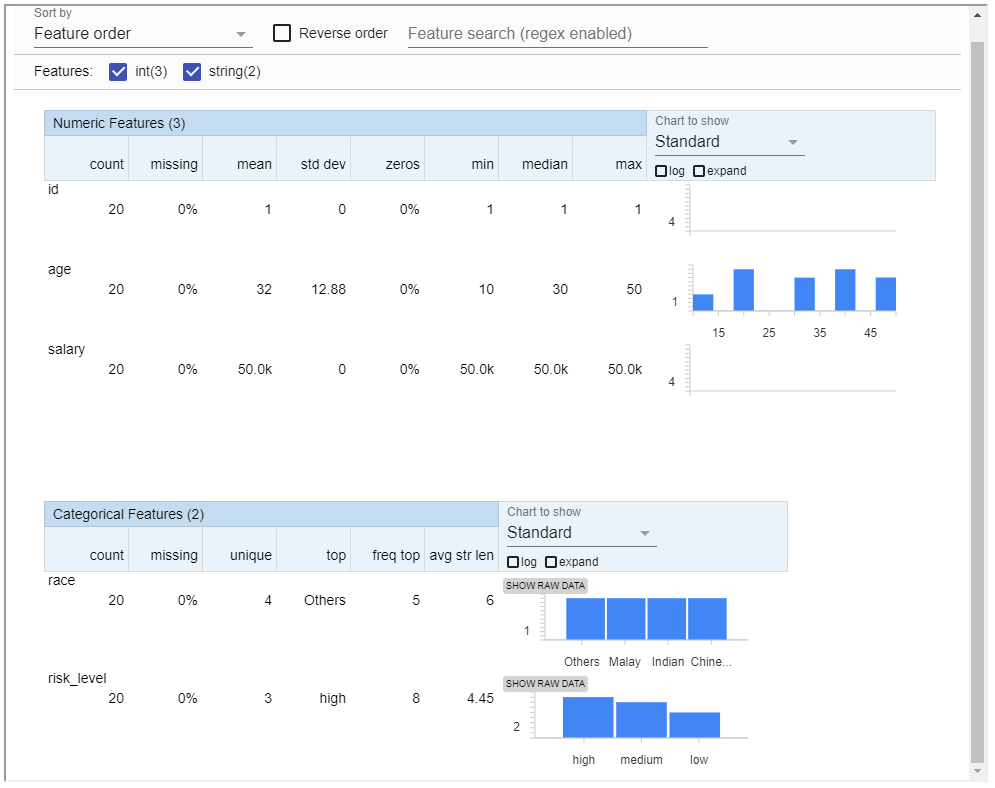Alternatively, generate statistic into text format.

1
tfdv.write_stats_text(my_train_stats, "my_train_stats.pbtext")


# Auto Schema Creation

After we have loaded the dataset (train.csv), we can extract schema from my_train_stats.

1
2
my_schema = tfdv.infer_schema(statistics=my_train_stats)
tfdv.display_schema(schema=my_schema)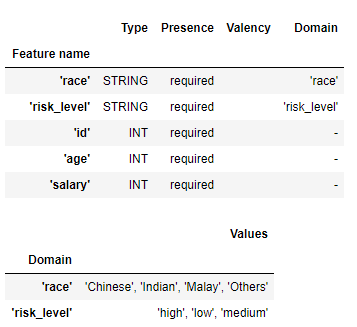Run the code below to generate schema into text format.

1
tfdv.write_schema_text(my_schema, "train_schema.pbtext")


# Validate anomalies

We can validate the a new dataset by using the schema object, my_schema created earlier.

1
2
3
4
5
6
# Import new dataset
my_train_missing_stats = tfdv.generate_statistics_from_csv(data_location="train-missing-field.csv")

# Validate
my_anomalies = tfdv.validate_statistics(statistics=my_train_missing_stats, schema=my_schema)
tfdv.display_anomalies(my_anomalies)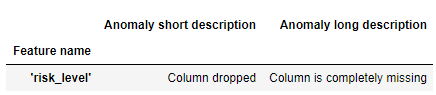Use the following code to ignore this anomalies.

1
2
3
4
5
6
7
8
9
10
11
12
# All features are by default in both TRAINING and SERVING environments.
my_schema.default_environment.append('TRAINING')
my_schema.default_environment.append('SERVING')

# Specify that 'risk_lvel' feature is not in SERVING environment.
tfdv.get_feature(my_schema, 'risk_level').not_in_environment.append('SERVING')

serving_anomalies_with_env = tfdv.validate_statistics(statistics=my_train_missing_stats,
schema=my_schema,
environment='SERVING')

tfdv.display_anomalies(serving_anomalies_with_env)


# Detect Data Skew

TFDV can detect data skew on training and serving dataset.

In our example below, we want to detect data skew that happened in feature race.

1
2
3
4
5
6
7
8
9
10
my_test_stats = tfdv.generate_statistics_from_csv(data_location="test.csv")

race=tfdv.get_feature(my_schema, 'race')
race.skew_comparator.infinity_norm.threshold = 0.1

my_skew_anomalies = tfdv.validate_statistics(my_train_stats, my_schema,
serving_statistics=my_test_stats)

tfdv.display_anomalies(my_skew_anomalies)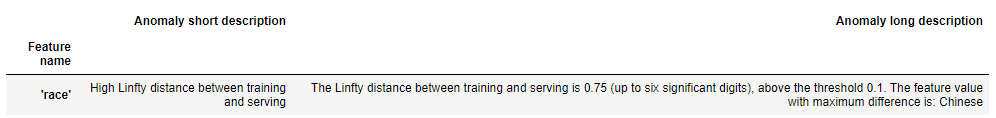# Detect Data Drift

TFDV can detect data drift (categorial attribute/feature) from multiple dataset.

In our example below, we want to detect data drift that happened in feature risk_level.

1
2
3
4
5
6
7
8
9
10
11
12
13
14
# Import new dataset
my_train2_stats = tfdv.generate_statistics_from_csv(data_location="train2.csv")

# Create new schema to prevent messing up with data skew example
my_schema2 = tfdv.infer_schema(statistics=my_train_stats)

risk_level=tfdv.get_feature(my_schema2, 'risk_level')
risk_level.drift_comparator.infinity_norm.threshold = 0.1

my_drift_anomalies = tfdv.validate_statistics(my_train_stats, my_schema2,
previous_statistics=my_train2_stats,
serving_statistics=my_test_stats)

tfdv.display_anomalies(my_drift_anomalies)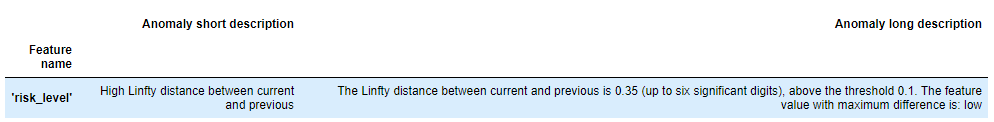# Conclusion

TFDV is really a handy tool that do exactly what it stand for and can be used individually even you’re not training model with Tensorflow.

Check out the code snippet and csv data here (GitHub).